top of page##### Hexagon grid building tool

This semester, I built a hexagon grid layout in Unity for our sandbox game, right here I want to share my approach to implementing the system.

##### How Unity Render Mesh

Unity engine uses a Rendering Pipeline to render meshes, which is responsible for managing the various stages involved in rendering 3D graphics, including mesh processing, lighting, shading, and post-processing.

When Unity renders a mesh, it first processes the mesh data to create an optimized representation of the mesh. This includes calculating the vertex positions, normal vectors, and texture coordinates, which are managed by a Mesh Filter component.

Once the mesh data has been processed, Unity applies lighting and shading to the mesh via a component called Mesh Renderer. This involves calculating the lighting and shadowing effects that are applied to the mesh based on the position and intensity of the light sources in the scene.##### Draw a hollow hexagon

To render a hollow hexagon, we can divide it into two parts: the outer ring and the inner ring. Vertices on the hexagon ring can be treated as every time a circle's radius rotates 60 degrees we mark a point. The point's position in Vector3 can be denoted as:Now we have the position of the vertices, let's take a look at how to draw a hollow hexagon segment without considering its thickness.

In Unity, every mesh is represented by a number of triangles. For example, each hollow hex segment can be treated as two triangles: △AiBoAo and △AiBiBo.To render a triangle, we need three lists that store the triangle's vertex position, its UVs, and the information on how the vertices are connected respectively.

The vertices list stores the involving vertices' positions in 3D space. The triangle list is a bit more tricky, it's an integer list with a size that can be evenly divided by 3 because every element is representing a vertex in the vertice array at that index, and every three elements represent how a triangle should be formed.

UV list is easier to understand, it should have a length that equals the length of the

vertices list. Each value defines the vertex in the vertices list that shares the same index

as the current UV value its UV position on the texture map. In the above example, we

are assigning pointAi's position on the texture map to be (0,0)Now we know how to render a triangle, let's try to move to something harder, such as a hexagon segment. Let's define a struct that stores the key information to render a hexagon segmentTake a look back at the hollow hexagon segment and take its thickness into consideration, we can see that each hex segment consists of 4 surfaces.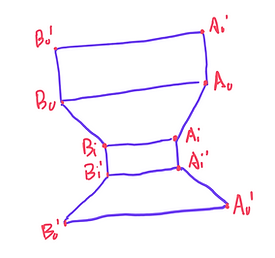Luckily, we can see all four faces are quadrilateral, which indicates that there can be a single method that can create a HexSegment object that stores the information of each face.

One thing worth mentioning is that, in Unity, the sequence of the vertices in the rendering vertices array matters because every triangle has a front face and a back face. Taking the right picture as an example, 012 is a counterclockwise rendering sequence, which will induce the triangle to be visible when we are looking at its back (because its front face is facing backward). If we flip the sequence to 210 we will make the triangle face forward.Above is the helper method I created for calculating relevant information about a face and putting them into a HexSegment data structure. Noticing that it takes a boolean as an input parameter because we need to determine whether the face should face forward by default or backward. With the help of this function, we can find all the required faces for a single hexagon with their key information stored in the HexSegment object.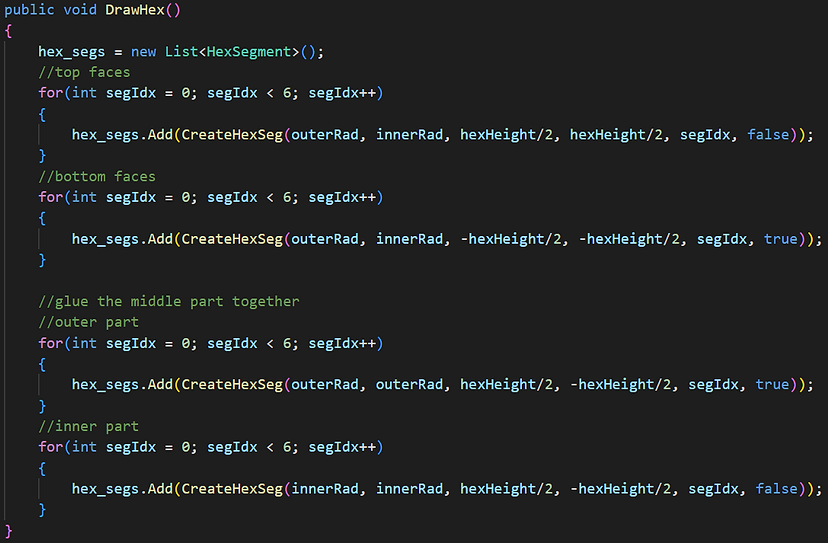Last but not least, we need to glue all the segments together by merging their vertice list, UV list, and triangle list.Result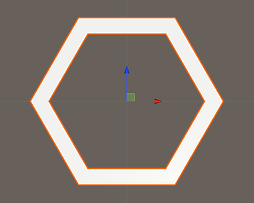##### Navigation On a Hexagon Layout

Once we've mastered the process of rendering a single hexagon, we can easily generate a full hexagonal layout. This can be achieved using a while loop, combined with precise manipulation of the positional offsets for each subsequent hexagon.

In addition, I developed a separate script, HexTile.cs, designed to function as a data container for each individual tile. This strategy was adopted to segregate the responsibilities of data management from the rendering functionality, thus enhancing modularity and code readability.To implement pathfinding within the hexagonal layout, it's crucial to have a mechanism for calculating the distance between any two arbitrary tiles. However, under our current offset coordinate system, this becomes a challenge.

Consider, for example, the tiles at positions (-1,0) and (-2, -1); they are neighboring tiles. Simultaneously, tiles at (-2, -1) and (-2, -2) are also neighbors. Given these circumstances, it becomes exceedingly difficult to discern whether two tiles are neighbors solely based on their offset positions

Another way to look at hexagonal grids is to see that there are three primary axes, unlike the two we have for square grids. There's an elegant symmetry with these.

Let's take a cube grid and slice out a diagonal plane at x + y + z = 0. This is a weird idea but it helps us with hex grid algorithms:

• 3d cartesian coordinates follow standard vector operations: we can add/subtract coordinates, multiply/divide by a scalar, etc. We can reuse these operations with hexagonal grids. Offset coordinates do not support these operations.

• 3d cartesian coordinates have existing algorithms like distances, rotation, reflection, line drawing, conversion to/from screen coordinates, etc. We can adapt these algorithms to work on hexagonal grids.Once we've transformed the grid's coordinates from offset space to cube space, determining the distance between any two arbitrary tiles becomes straightforward. We can simply apply the distance formula typically used in 3D Cartesian coordinates.

Besides, moving one space in hex coordinates involves changing one of the 3 cube coordinates by +1 and changing another one by -1 (the sum must remain 0). There are 3 possible coordinates to change by +1, and 2 remaining that could be changed by -1. This results in 6 possible changes and each corresponds to one of the hexagonal directions: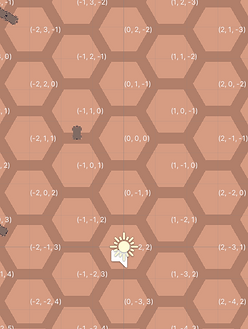Now that we have completed all the necessary groundwork, we are ready to implement pathfinding algorithms on our tiles!

I opted to utilize the A* algorithm due to its superior speed when compared to traditional Dijkstra algorithm methods.

The A* algorithm works by using a heuristic to estimate the cost (distance) from each node to the goal, in addition to the actual cost from the start node to each node. This allows it to prioritize nodes that are likely to lead to the goal more quickly. It's important to note that all heuristic-related calculations necessitate the conversion of tiles to cube coordinate positions, enabling distance computation under 3D Cartesian coordinates.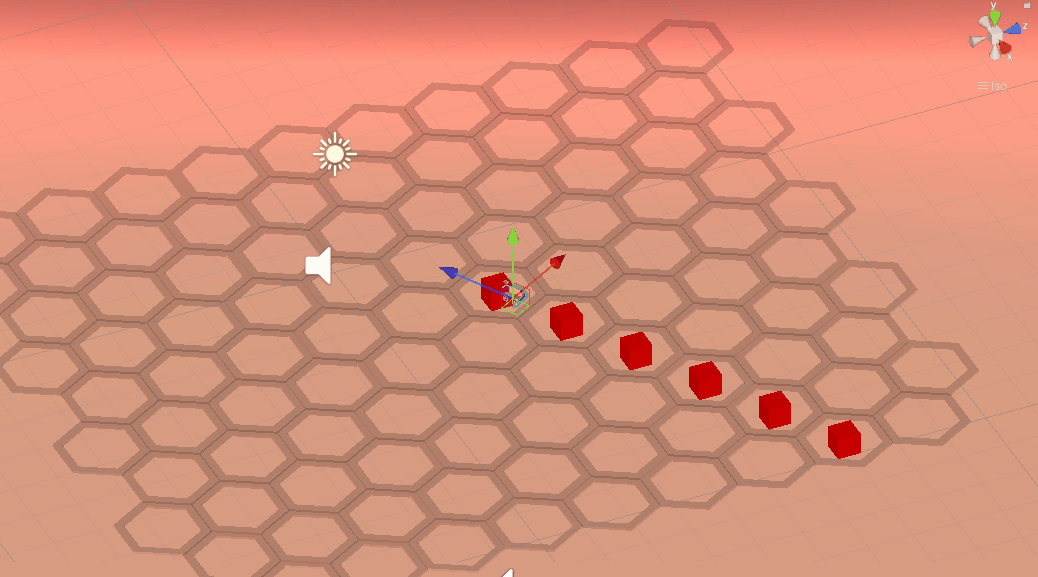Final result

bottom of page